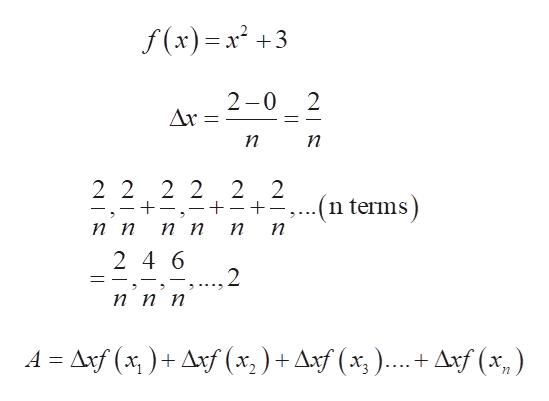# For the function given​ below, find a formula for the Riemann sum obtained by dividing the interval ​[00​,22​] into n equal subintervals and using the​ right-hand endpoint for each c Subscript kck. Then take a limit of this sum as n right arrow infinityn → ∞ to calculate the area under the curve over ​[00​,22​].f left parenthesis x right parenthesis equals x squared plus 3f(x)=x2+3Write a formula for a Riemann sum for the function f left parenthesis x right parenthesis equals x squared plus 3f(x)=x2+3 over the interval ​[00​,22​].

Question
1 views

For the function given​ below, find a formula for the Riemann sum obtained by dividing the interval ​[
0
0​,
2
2​] into n equal subintervals and using the​ right-hand endpoint for each
c Subscript k
ck. Then take a limit of this sum as
n right arrow infinity
n → ∞ to calculate the area under the curve over ​[
0
0​,
2
2​].

f left parenthesis x right parenthesis equals x squared plus 3
f(x)=x2+3
Write a formula for a Riemann sum for the function
f left parenthesis x right parenthesis equals x squared plus 3
f(x)=x2+3 over the interval ​[
0
0​,
2
2​].

check_circle

Step 1

Given the interval [0,2] is to be divided into n subintervals and right-hand endpoint for each interval is to be used. The function is:

Dividing the interval into subintervals:

So the right end points are given by:

The Riemann Sum for a function is given by:

Where x1, x2, x3… are the endpoints considered.help_outlineImage Transcriptionclosef(x) x +3 2-0 2 п п 2 2 2 2 2 2 +-+ (n terms) пп пп п 246 2 _ ппп = Arf (x)+Axf (x, ) + Axf (x, ).+ Axf (x,) + fullscreen
Step 2

Using the above formula, the R...

### Want to see the full answer?

See Solution

#### Want to see this answer and more?

Solutions are written by subject experts who are available 24/7. Questions are typically answered within 1 hour.*

See Solution
*Response times may vary by subject and question.
Tagged in

### Integration